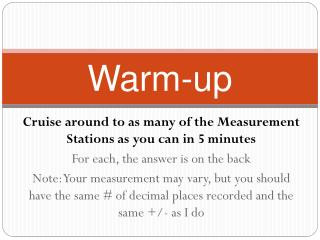DownloadDownload PresentationWarm-up

# Warm-up

Download Presentation## Warm-up

- - - - - - - - - - - - - - - - - - - - - - - - - - - E N D - - - - - - - - - - - - - - - - - - - - - - - - - - -
##### Presentation Transcript

1. Warm-up Cruise around to as many of the Measurement Stations as you can in 5 minutes For each, the answer is on the back Note: Your measurement may vary, but you should have the same # of decimal places recorded and the same +/- as I do

2. Uncertainties How to find the +/- uncertainty of measured and calculated values IB Chemistry

3. Significant Figures • Since you work hard to be accurate, it’s important that you show off just how careful you were with your measurements. • Examples: 76.0cm3 52.8cm3 6.59cm3

4. Significant Figures • Significant Figures are values that have been measured (and deserve respect) • 1.98 = 3 sig figs Numbers are significant • 2 = 1 sig fig • 2.05 = 3 sig figs Zeros are sig. if between numbers • 2.00 = 3 sig figs Zeros are sig. if behind decimal • 0.0002 = 1 sig fig … and not at the beginning • 2000 = 1 sig fig (but, 2000. = 4 sig figs) Zeros at the end are not significant • 2.000 x 103 = 4 sig figs Everything is sig. in scientific notation • Adding and subtracting- keep the same # of digits after the decimal as the value with the least #s after decimal. • Ex: 2.3 + 1.444 = 2.744 = 2.7 • Multiplying and Dividing- keep the same # of sig figs as the value with the least • Ex: 0.002 x 3.897 = 0.007794 = 0.008 Note: Just worry about these at the end and they’re really not too bad.

5. What is “Uncertainty”? • Every time we measure a value, there’s a certain point where we (or the instrument that we’re using) have to estimate or round • So, no measurement is absolutely perfect • The imperfectness is the uncertainty • This can be expressed with a ± value at the end • Example: 2.5cm ± 0.5 means that the actual value is 2.0 – 3.0 2.50cm ± 0.05 means 2.45 - 2.55 The second value is more precise (note: uncertainties only have 1 significant figure)

6. Finding Uncertainty- Measured • Analog equipment: ± HALF of the increment on the instrument, but you could use your judgment here, with a short justification statement • Ex: 76.0cm3± 0.5 (or even 76.0cm3± 0.2 with justification) • Ex: 6.55cm3± 0.05 • Digital equipment: ± WHOLE last digit measured • Ex: 1.25g ± 0.01g • Ex: pH= 6.5 ± 0.1

7. Try some… • Measuring mass on a digital balance: • 0.34g • 1.9998g • Measuring temperature with digital thermometer: • 24oC • 19.6oC • Measuring temp with a manual thermometer: • 14.5oC • Measuring volume with a burette: • 34.5cm3 ± 0.01 ± 0.0001 ± 1 ± 0.1 ± 0.5 ± 0.5

8. Finding Uncertainty- Calculated I (Propagation of Error) Uncertainties ALWAYS have only 1 significant figure • Adding and Subtracting: • Add uncertainties of all values used • Ex: • Volume change = 45.60 ±0.05 cm3– 34.10 ± 0.05 cm3 • Could be as small as 45.55 – 34.15 = 11.40 • Could be as large as 45.65 – 34.05 = 11.60 • So… = 11.50 ± 0.1 • Ex #2: • Mass change = 0.8 ± 0.1 g – 0.7 ± 0.1 g = 0.1 ± 0.2 • Averaging: • Half of the range of values averaged, or the ±, whichever is bigger • Ex: • average these values: 3.4, 2.6, 3.2 (all ± 0.1) • 3.1 ± 0.1 0.4

9. Try Some- Addition/Subtraction • (45 ± 1)+(23 ± 1) = • (0.9 ± 0.1)+(0.8 ± 0.1)–(0.5 ± 0.1) = • Average: 9.1 ± 0.1, 9.5 ± 0.1, and 9.2 ± 0.1 = 68 ± 2 1.2 ± 0.3 9.2 ± 0.2

10. Finding Uncertainty- Calculated II • Multiplying and Dividing • Convert all uncertainties into percents • Add the percents • Convert the percent back into an absolute uncertainty • Ex: • Density = (4.5g ± 0.1)/(9cm3± 1) • 0.1 is 2.2% of 4.5 • 1 is 11.1% of 9 • Density = 0.5g/cm3± 13.3% • 13.3% of 0.5 is 0.07 • Answer… Density = 0.5 ± 0.07 Don’t forget significant figures at the end

11. Try Some– Multiplication/Division • Rate = (23.9m ± 0.1)/(134sec ± 1) • % Water = (0.049g ± 0.001)/(0.092 ± 0.001) • 0.1 = 0.42%, 1 = 0.75% • Rate = 0.178m/sec ± 1.17% • Rate = 0.178m/sec ± 0.002 • 0.001 = 2.0%, 0.001 = 1.1% • % Water = 53% ±3.1% of 53 • % Water = 53% ± 2

12. A Quickie Way • Usually, the range of your data is much larger than the propagated uncertainty • Calculate the final value using your trials, average your trials and then add a ± using ½ of the range to your final answer… • This could save you a lot of converting to percents…. blah blah blah along the way • Example:

13. Example– Quickie way • 3 values are calculated using the cumbersome % method: • 43.56 ± 0.04 • 40.99 ± 0.02 • 44.03 ± 0.03 • Average= 42.86 • Uncertainty is half of the range or the uncertainty of the value whichever is larger • The range is 44.03-40.99= 3.04 • half of which is 1.52 or 2 with one sig fig • 2 is huge compared to the uncertainties of the values, so why did you bother with all that mess? Just skip it! Answer = 42.86 ± 2